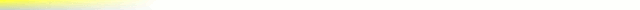# [mkgmap-dev] comparing numeric values

From Torsten Leistikow de_muur at gmx.de on Wed Jul 29 19:03:34 BST 2009

```Moin,

in the style rules documentation in the WIKI it is stated, that a
comparison of numeric quantities is possible, e.g. "maxspeed>=30".

Is this really working? I tried the following example:

In my osm file I had five ways:
<way id='-9902' action='modify' visible='true'>
<nd ref='-9900' />
<nd ref='-9901' />
<tag k='highway' v='null_null' />
<tag k='layer' v='-1' />
<tag k='name' v='layer=-1' />
</way>

<way id='-9912' action='modify' visible='true'>
<nd ref='-9910' />
<nd ref='-9911' />
<tag k='highway' v='null_null' />
<tag k='layer' v='0' />
<tag k='name' v='layer=0' />
</way>

<way id='-9922' action='modify' visible='true'>
<nd ref='-9920' />
<nd ref='-9921' />
<tag k='highway' v='null_null' />
<tag k='name' v='layer!=*' />
</way>

<way id='-9932' action='modify' visible='true'>
<nd ref='-9930' />
<nd ref='-9931' />
<tag k='highway' v='null_null' />
<tag k='layer' v='1' />
<tag k='name' v='layer=1' />
</way>

<way id='-9952' action='modify' visible='true'>
<nd ref='-9950' />
<nd ref='-9951' />
<tag k='highway' v='null_null' />
<tag k='layer' v='+1' />
<tag k='name' v='layer=+1' />
</way>

And in my style the lines-file contained the following rules:
highway=null_null & layer<0  [0x01 resolution 10]
highway=null_null & layer=0  [0x02 resolution 10]
highway=null_null & layer>0  [0x03 resolution 10]
highway=null_null & layer='-1'  [0x04 resolution 10]
highway=null_null & layer='0'  [0x05 resolution 10]
highway=null_null & layer='1'  [0x06 resolution 10]
highway=null_null & layer='+1'  [0x07 resolution 10]
highway=null_null   [0x08 resolution 10]

As a result I expected the following conversions
9902 -> 0x01
9912 -> 0x02
9922 -> 0x08
9932 -> 0x03
9952 -> 0x03

Against my expectations the conversions were the following:
9902 -> 0x03
9912 -> 0x05
9922 -> 0x08
9932 -> 0x03
9952 -> 0x03

What is wrong?
- My understanding of the style rules?
- The documentation of the style rules?
- The behaviour of mkgmap?

Gruss
Torsten

```